Next: Derivation of Similarity Function Up: Problem Formulation Previous: Example

## Probabilistic Forms

Likelihood is: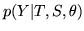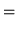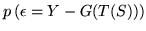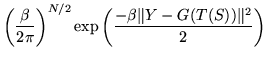Priors: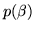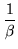For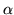there are two alternative priors that are useful.

1. Flat prior: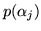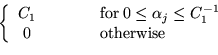where the range of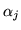is restricted to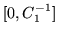.
2. Or a prior encoding prior knowledge: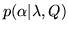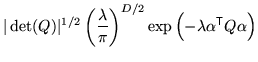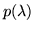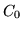where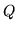represents the prior knowledge about the expected intensities in the image formation;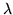is a parameter expressing the unknown scaling between the learnt prior distribution ofparameters and the intensities in a new image; andis a constant, representing the (improper) flat prior on.

Parameters: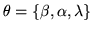where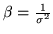is the precision parameter.Next: Derivation of Similarity Function Up: Problem Formulation Previous: Example